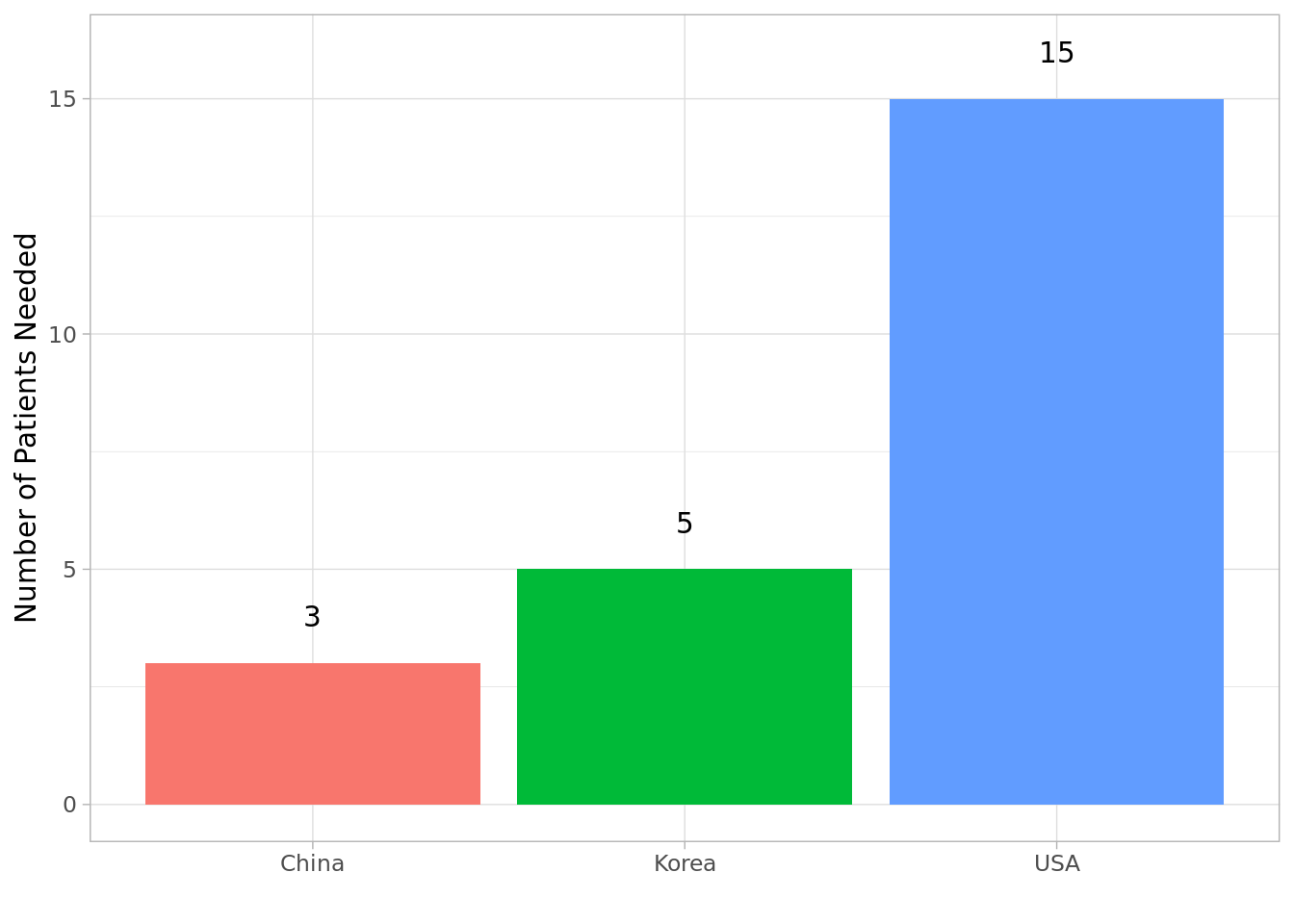# Sample Size Caluclation in R… Extended!

This is an extension from a post I saw here. When thinking about study designs for a trial that we want to conduct for a client, one of the things that we need to think about is how many patients we would need to recruit to see the effect that we propose to see? We’re going to walk through a couple of scenarios and see how we need can use R to get at the sample size & power question when desgining our studies.

# Scenario 1: One Sample Mean T-Test

There aren’t many scenarios where we want to test one population and if they have a significantly differing values of a parameter of interest from a null value. But let’s start with an easy example first.

Let’s say we’re interested determining if the average hospital cost of patients with hemophilia is less than $80,000 a year. We collect trial data. The data is shown below. df_s1 <- data.frame( cost_hosp = c(70000,80000,2000,13000,140000,13000,24000,44000,87000,30000) ) # load library to summarize data library('pacman') p_load(skimr,dplyr) df_s1 %>% skim()  Name Piped data Number of rows 10 Number of columns 1 _______________________ Column type frequency: numeric 1 ________________________ Group variables None Variable type: numeric skim_variable n_missing complete_rate mean sd p0 p25 p50 p75 p100 hist cost_hosp 0 1 50300 43361.66 2000 15750 37000 77500 140000 ▇▃▃▂▂ Now that we have a sense of the data, we need to extract the effect size in order to estimate the number of patients we need to survey/collect for our study. The equation for effect size is as below $\text{effect size} = \frac{\text{mean}_{\text{sample}} - \text{mean}_{\text{hypothesized}}}{\text{standard deviation}_{\text{sample}}}$ Below is the R code for calculating the effect size effsize <- (mean(df_s1$cost_hosp)  - 80000) / sd(df_s1$cost_hosp) effsize ##  -0.6849369 Now we can use the {pwr} package to estimate the sample size needed. We’re going to assume an of 0.05 and power of 0.80. The hypothesis of interest is whether the estimated cost from our sample is smaller than$80,000.

p_load(pwr)
pwr.t.test(d = effsize, sig.level =0.05, power=0.80, type = "one.sample", alternative= "less")
##
##      One-sample t test power calculation
##
##               n = 14.63024
##               d = -0.6849369
##       sig.level = 0.05
##           power = 0.8
##     alternative = less

Given that we see the number going over 14, we need to round up and collect information from 15 patients.

Now let’s say we’re interested in instances where different countries have different mean costs and therefore we’d be interested to see how our sample sizes need to pan out in different countries. See the data below:

df_s1b <- data.frame(
cost_hosp_usa = c(70000,80000,2000,13000,140000,13000,24000,44000,87000,30000),
cost_hosp_china = c(20000,24000,2300,15000,40000,6300,9400,10000,27000,23000),
cost_hosp_korea = c(30000,40000,3000,10000,100000,3500,14000,23000,87000,30000)
)

df_s1b %>%
skim()
 Name Piped data Number of rows 10 Number of columns 3 _______________________ Column type frequency: numeric 3 ________________________ Group variables None

Variable type: numeric

skim_variable n_missing complete_rate mean sd p0 p25 p50 p75 p100 hist
cost_hosp_usa 0 1 50300 43361.66 2000 15750 37000 77500 140000 ▇▃▃▂▂
cost_hosp_china 0 1 17700 11350.18 2300 9550 17500 23750 40000 ▇▅▇▂▂
cost_hosp_korea 0 1 34050 33700.02 3000 11000 26500 37500 100000 ▇▇▁▁▃

Let’s create a effect size df now

effsizedf <- data.frame(
es = c((mean(df_s1b$cost_hosp_usa) - 80000) / sd(df_s1b$cost_hosp_usa) , (mean(df_s1b$cost_hosp_china) - 80000) / sd(df_s1b$cost_hosp_china), (mean(df_s1b$cost_hosp_korea) - 80000) / sd(df_s1b$cost_hosp_korea)),
country = c("USA","China","Korea")
)
effsizedf
##           es country
## 1 -0.6849369     USA
## 2 -5.4888980   China
## 3 -1.3635005   Korea

Using the effsizedf we’re going to create another data.frame that will give us the estimated sample size to arrive at the effect estimate. We’re going to use{purrr} to apply the pwr.t.test function across the rows of the effsizedf.

p_load(purrr)

ss_s1 <- effsizedf %>%
pmap_dfr(function(es, country){
data.frame(
country = country,
es = es,
n = ceiling(pwr.t.test(d = es, sig.level =0.05, power=0.80, type = "one.sample", alternative= "less")\$n)
)
})
ss_s1
##   country         es  n
## 1     USA -0.6849369 15
## 2   China -5.4888980  3
## 3   Korea -1.3635005  5

To make it easier on the eyes let’s visualize it using {ggplot2}.

p_load(ggplot2)

p<-ggplot(data=ss_s1, aes(x=country, y=n, fill=country, label=n)) +
geom_bar(stat="identity") +
geom_text(nudge_y = 1) +
theme_light() +
theme(legend.position = "none") +
xlab("") + ylab("Number of Patients Needed")
pSo there you have it. As the importance of real-world evidence is continuing to grow and emphasis on generating value continues to rise, I hope more people will be able to utilize codes written in R (or python or SAS) when designing real-world studies.

I’ll be editing this post from time to time to add more power & sample size calculations for different tests. Thank you for reading and happy coding!##### Chong H. Kim
###### Health Economics & Outcomes Researcher

My research interests include real-world evidence/observation research, cost-effectiveness analysis, predictive modeling, and spatial statistics.